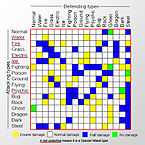Want to share your content on R-bloggers? click here if you have a blog, or here if you don't.The table() function is intended for use during the Data Exploration phase of Data Analysis. The table() function performs categorical tabulation of data. In the R programming language, “categorical” variables are also called “factor” variables.

The tabulation of data categories allows for Cross-Validation of data. Thereby, finding possible flaws within a dataset, or possible flaws within the processes used to create the dataset. The table() function allows for logical parameters to modify data tabulation.

Beyond Data Exploration, the table() function allows for the inference of statistics within multivariate tables, (or contingency tables), of two or more variables.

Answers to the exercises are available here.

Exercise 1

Basic tabulation of categorical data

This is the first dataset to explore:
`Gender <- c("Female","Female","Male","Male") Restaurant <- c("Yes","No","Yes","No") Count <- c(220, 780, 400, 600) DiningSurvey <- data.frame(Gender, Restaurant, Count) DiningSurvey`

Using the table() function, compare the Gender and Restaurant variables in the above dataset.

Exercise 2

The table() function modified with a logical vector.

Use the logical vector of “Count > 650” to summarize the data.

Exercise 3

The useNA & is.na arguments find missing values.

First append the dataset with missing values:
`DiningSurvey\$Restaurant <- c("Yes", "No", "Yes", NA)`

Apply the “useNA” argument to find missing Restaurant data.

Next, apply the “is.na()” argument to find missing Restaurant data by Gender.

Exercise 4

The “exclude =” parameter excludes columns of data.

Exclude one of the dataset’s Genders with the “exclude” argument.

Exercise 5

The “margin.table()” function requires data in array form, and generates tables of marginal frequencies. The margin.table() function summarizes arrays within a given index.

First, generate array format data:
`RentalUnits <- matrix(c(45,37,34,10,15,12,24,18,19),ncol=3,byrow=TRUE) colnames(RentalUnits) <- c("Section1","Section2","Section3") rownames(RentalUnits) <- c("Rented","Vacant","Reserved") RentalUnits <- as.table(RentalUnits)`

Find the amount of Occupancy summed over Sections.

Next, find the amount of Units summed by Section.

Exercise 6

The prop.table() function creates tables of proportions within the dataset.

Use the “prop.table() function to create a basic table of proportions.

Next, find row percentages, and column percentages.

Exercise 7

The ftable() function generates multidimensional n-way tables, or “flat” contingency tables.

Use the ftable() function to summarize the dataset, “RentalUnits”.

Exercise 8

The “summary() function performs an independence test of the dataset’s factors.

Use “summary()” to perform a Chi-Square Test of Independence.

Exercise 9

“as.data.frame()” summarizes frequencies of data arrays.

Use “as.data.frame()” to list frequencies within the “RentalUnits” array.

Exercise 10

The “addmargins()” function creates arbitrary margins on multivariate arrays.

Use “addmargins()” to append “RentalUnits” with sums.

Next, summarize columns with “RentalUnits”.

Next, summarize rows with “RentalUnits”.

Finally, combine “addmargins()” and “prop.table()” to summarize proportions within “RentalUnits”. What is statistically inferred about sales of rental units by section?

Image by by IngerAlHaosului.